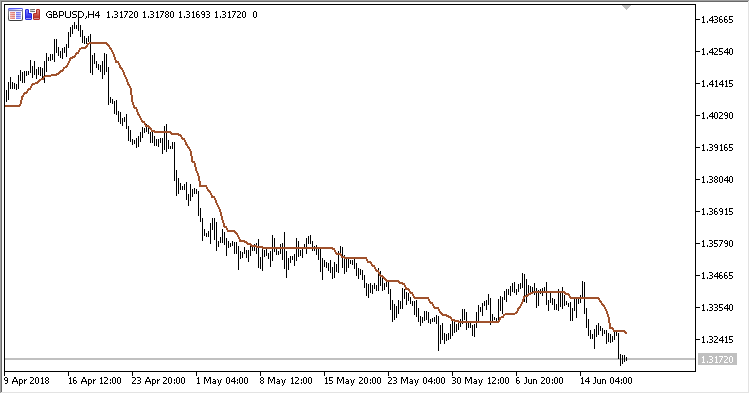Interesting script?
So post a link to it -
let others appraise it

You liked the script? Try it in the MetaTrader 5 terminal# CA - indicator for MetaTrader 5

Views:
901
Rating:
Published:
2018.07.13 15:19

Indicator CA (Corrected Average), also known as Optimal Moving Average. The benefit of the indicator is the fact that the current value of the timeseries must exceed the current threshold that depends on volatility for the indicator line to follow the price, avoiding false signals in the trend.

The indicator has three configurable parameters:

• Period - calculation period;
• Method - calculation method;
• Applied price - price used for calculations.

Calculations:

`CA = PrevCA + k * (MA-PrevCA)`

where:

`MA - MA(Applied price, Period, Method)`

If diff2 > Dev:

`k = 1-Dev / diff2`

otherwise:

`k = 0`
```Dev = StdDev*StdDev
StdDev - Standard deviation(Applied price,Period)
diff2 = diff * diff,
diff = PrevCA - MA
```Translated from Russian by MetaQuotes Software Corp.
Original code: https://www.mql5.com/ru/code/21160ASO

Indicator ASO (Average Sentiment Oscillator) displays the general market climate.SymbolX_Candle

This indicator calculates the index of a given currency, using the USD index. It is based on indicator SymbolX, but four prices are used for calculations: OHLC instead of just one Close price.DMX

Indicator DMX (Bipolar DMI) is calculated using the standard indicator ADX. However, as compared to that, it displays data as an oscillator with a signal line, and has shorter delay.GB

Indicator GB (Gaussian Bands) works in the same manner as standard indicator Bollinger Bands. However, unlike the former, it performs filtering centerline and deviation lines by Gaussian method. It also has two deviation lines on each side of the centerline.